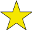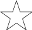## Number Crossword #1

Difficulty:(explanation of difficulty)

Instructions: Fill in each white-coloured box in the grid with a single numeral between 0 and 9, corresponding with the digits in each answer. No number begins with the digit 0. When finished, select the "Check solution" button to check your solution.

When a letter is entered into a square: Do nothing Move cursor right Move cursor down

 1 2 3 4 5 6 7 8 9

### Across

1. 13 + 53 + 33.
2. The smallest number that can be written as a sum of cubes in two different ways.
3. A fifth power.
4. A square number.
5. 2 down + 4 down − 8 down.
6. A square number.

### Down

1. The seventh prime number.
2. The thousands digit minus the hundreds digit equals the tens digit in this multiple of ten.
3. 22 × 18.
4. Four digits in ascending order.
5. One more than the number of degrees in a circle.
6. A multiple of 21.